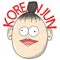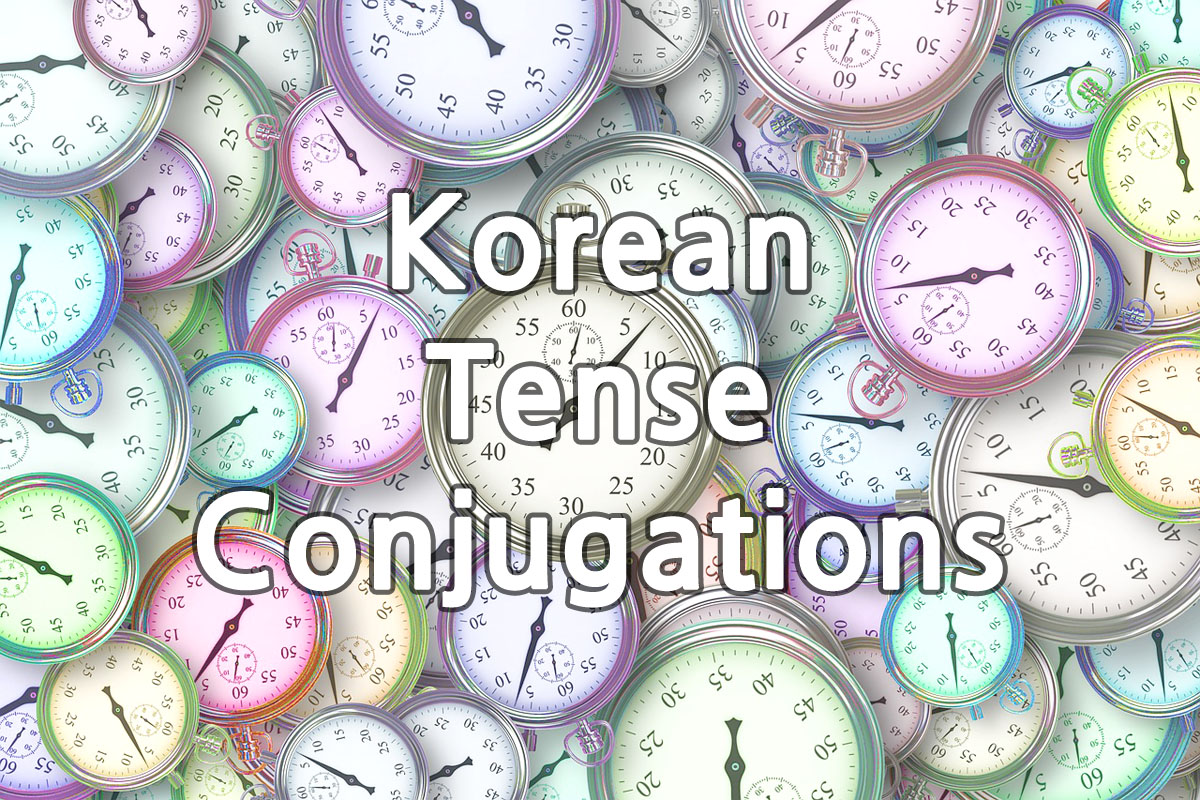# 11. Tense Conjugations

Korean grammar uses conjugations to decide the tense of sentence, just like other languages do. You’ve learned that in a previous lesson and you will learn exactly which part decides it and how in this lesson.You will learn how Korean tense conjugation system works in this lesson, not tenses. You will learn each tense after this. Don’t waste your time to memorize each tense conjugations for now. Normally Korean textbooks and teachers don’t teach how it really works. That’s okay at the first but it means only confusion later. That’s why I want you to understand how it works.

## The Most Basic Form

Korean language doesn’t have a particular basic form so it uses the present tense as the most basic conjugated form. Always remember the basic form uses present tense.

## Tense

The tense of sentence is always decided before the ending by changing the tense conjugation. That’s most important point. Ending is the speech style such as 다, 요, 습니다.

Now I’ll show you examples using 3 tense conjugations: present, past and future. Check how the word changes when using each one.

다 = Do (Basic form)
다 = Did
할 것이다 = Will do

노래다 = Sing (Basic form)
노래다 = Sang
노래할 것이다 = Will sing

다 = Am / Are / Is (Basic form)
다 = Was / Were
을 것이다 = Will be

감자가 다 = There is a potato (Basic form)
감자가 있다 = There was a potato
감자가 있을 것이다 = There will be a potato (it sounds like LET THERE BE POTATO!)
있을 것이다 sounds so serious with everything. Super serious.

You can see the tense of the sentence changes before the ending ‘다. Tense is ALWAYS decided before the ending.

It’s a really important lesson. I’ve seen many students just learn many conjugations and phrases without understanding how they work. It might be okay at first time but it slows you down and make it difficult for you to speak in real conversations.

## Why Do I Need To Know This?

Korean has so many speech styles but mostly those tense conjugations don’t change. Now you’re learning 해요 speech style in this course. so I’ll show you some examples with 해요 speech style and you can see the same tense rules and conjugations applied to other speech styles.

### 해요 speech style

요 = Do (Basic form)
어요 = Did
할 것이예요 = Will do

노래요 = Sing (Basic form)
노래어요 = Sang
노래할 것이예요 = Will sing

### 합니다 speech style

습니다 = Am / Are / Is (Basic form)
습니다 = Was / Were
을 것입니다 = Will be

감자가 습니다 = There is a potato (Basic form)
감자가 있니다 = There was a potato
감자가 있을 것입니다 = There will be a potato

## Another Reason

in previous lessons somewhere, I taught you that Korean conjugations can be combined. Let’s say you have to say ‘I could’, Then most students try to learn a new phrase for ‘could’. However, Korean grammar has so many conjugations, like hundreds, it would be thousands if you have to memorize them all separately.

할 수 다 = Can

• 할 수 = possibility to do in the future
• 있었다 = Was /Were
• 을 것이다 = Will

할 수 있다 = Could
할 수 있을 것이다 = Will be able to

해야다 = have to

• 해야 = Variation of 하다
• 한다 = Variation of 하다
• 했다 = Did
• ㄹ 것이다 = Will